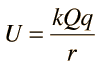# Electric Potential Energy

Potential energy can be defined as the capacity for doing work which arises from position or configuration. In the electrical case, a charge will exert a force on any other charge and potential energy arises from any collection of charges. For example, if a positive charge Q is fixed at some point in space, any other positive charge which is brought close to it will experience a repulsive force and will therefore have potential energy. The potential energy of a test charge q in the vicinity of this source charge will be:Show

where k is Coulomb's constant.

In electricity, it is usually more convenient to use the electric potential energy per unit charge, just called electric potential or voltage.

 Application:Coulomb barrier for nuclear fusion
 Energy in electron volts
Index

Voltage concepts

 HyperPhysics***** Electricity and Magnetism R Nave
Go Back

# Zero Potential

The nature of potential is that the zero point is arbitrary; it can be set like the origin of a coordinate system. That is not to say that it is insignificant; once the zero of potential is set, then every value of potential is measured with respect to that zero. Another way of saying it is that it is the change in potential which has physical significance. The zero of electric potential (voltage) is set for convenience, but there is usually some physical or geometric logic to the choice of the zero point. For a single point charge or localized collection of charges, it is logical to set the zero point at infinity. But for an infinite line charge, that is not a logical choice, since the local values of potential would go to infinity. For practical electrical circuits, the earth or ground potential is usually taken to be zero and everything is referenced to the earth.

 Zero of potential at infinity
 Zero of mechanical potential energy
Index

Voltage concepts

 HyperPhysics***** Electricity and Magnetism R Nave
Go Back

# Potential Reference at Infinity

The general expression for the electric potential as a result of a point charge Q can be obtained by referencing to a zero of potential at infinity. The expression for the potential difference is:Taking the limit as rb→∞ gives simplyfor any arbitrary value of r. The choice of potential equal to zero at infinity is an arbitrary one, but is logical in this case because the electric field and force approach zero there. The electric potential energy for a charge q at r is thenwhere k is Coulomb's constant.

Index

Voltage concepts

 HyperPhysics***** Electricity and Magnetism R Nave
Go Back